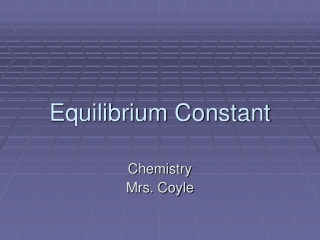DownloadDownload PresentationEquilibrium Constant

# Equilibrium Constant

Télécharger la présentation## Equilibrium Constant

- - - - - - - - - - - - - - - - - - - - - - - - - - - E N D - - - - - - - - - - - - - - - - - - - - - - - - - - -
##### Presentation Transcript

1. Equilibrium Constant Chemistry Mrs. Coyle

2. Equilibrium Position http://www.chm.davidson.edu/ronutt/che115/K/Sol_1.gif

3. Equilibrium Expression Equilibrium Constant, Keq • The concentrations are at the equilibrium position. • The Keq is constant for a given temperature regardless of the initial concentrations.

4. Keq > 1, products favored in equilibrium • Keq < 1, reactants favored in equilibrium • Keq is not expressed with units.

5. Rules for Keq of Heterogeneous Equilibrium (more than one phase) • Only gases and solutes concentrations appear in the equilibrium expressions. • Pure liquids and solids do not affect the Keq, because they do not change.

6. Practice • 2 SO2(g) + O2(g)⇄ 2 SO3(g)Keq= • AgCl(s) ⇄ Ag+ (aq) +Cl-(aq)Keq=

7. Ex. 1: Calculate the Keq , given equilibrium concentrations N2O4 (g)⇄ 2 NO2(g) At equilibrium at 10°C, [N2O4]= 0.0045 M and [NO2] = 0.030M.Calculate the Keq. Answer: Keq = 0.20